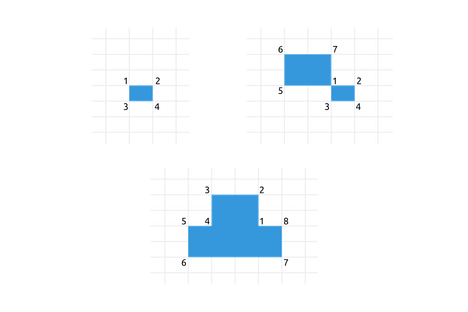# Putting GuardssamaunDIU Team Practice Contest...
Limits 1s, 1.0 GB

Shetu is playing a popular game called "Putting Guards". In this game there are various types of rectangular warehouses. The player has to calculate the minimum number of guards required to protect those. To protect those warehouses, each corner should have a guard. Shared corners should be covered by one guard. The whole space of the game is a 2D grid. The following figures explains some shapes of warehouses and necessary guards for them.## Input

Input begins with a single positive integer $T$ ($T < 30$) on a line which indicates the number of the cases. Each test case will start with 2 integers $R$ ($1≤ R ≤ 20$) and $C$ ($1 ≤ C ≤ 20$) on a line. $R$ is the number of rows and $C$ is the number of columns of the grid. Then $R$ lines follows with $C$ characters each. Each character is either 0 or 1. 1 means a cell containing warehouse body and 0 means a blank cell.

## Output

For each test case, the output must contain the case number and the total number of guards required like sample output.

## Sample

InputOutput
2
4 6
000000
000000
000011
000011
8 14
00000000000000
00000011000000
00000011000000
00011111111000
00011111111000
00000011000000
00000011000000
00000000000000

Case 1: 4
Case 2: 12ImplementationuDebug

### Submit